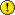help:chisquare

# The Chi Square Testif you have summary results already tallied, you can enter these directly into the On-line Chi-Square Calculator. SOFA runs off raw data only and creates all the group totals for you.

We use the Chi Square Test Pearson's chi-square test when investigating if there is a relationship between two categorical variables e.g. gender and age group.

The Chi Square Test works out how likely the observed level of relationship between two categorical variables would be by chance alone. If it is really unlikely, we reject the idea that the two are independent (we reject our null hypothesis). Be aware that there is a certain sensitivity about terminology around this area. According to a widespread convention, we shouldn't conclude that there is a relationship, only that we reject the null hypothesis (see Hypothesis testing). We might go so far as to reject the null hypothesis in favour of the alternative hypothesis. See Statistical hypothesis testing.

In addition to the actual test statistics and p value, it is useful to look at a contingency table with both expected and observed values. The expected values are what you would expect if there were no relationship at all - i.e. if both variables were completely independent. The observed values are what is actually in the data. If the observed values are very close to the values expected if there is no relationship, we conclude there is no evidence of a relationship. NB this doesn't mean there is no relationship, only that we have not found evidence for one. We never affirm the null hypothesis, we simply fail to reject it. Perhaps there is a relationship but our sample size was too small to detect it with confidence.

In the example below (NB false data for illustration only), the expected and observed values are very similar so we should not be surprised that the p value is high. A p value of approximately 0.5 suggests that we would get the level of difference observed, or greater, half the time on average by chance alone.

SOFA Statistics also produces clustered bar charts to display the relationship (or lack thereof). See how the pattern in the (false) data is much the same for males and females. There were differences, but only as much as would happen by chance about half the time.

If there are bigger differences, the p value will be much smaller because the differences are much less likely to happen by mere chance. In this example, the p value is effectively zero indicating that we can reject the null hypothesis with confidence in favour of the alternative hypothesis - namely, that there is a relationship between country and age groups (NB false data for illustration only).

 The variables might be ordinal but we are treating them as merely categorical - as if the order is not relevant.A video is available showing how to do the Chi-Squared test using SOFA Statistics: https://www.youtube.com/watch?v=tr1u-OuT0Ow

help/chisquare.txt · Last modified: 2012/08/12 18:16 (external edit)

### Page Tools